Courses

# Chemical Kinetics MCQ - 2 (Advanced)

## 31 Questions MCQ Test Chemistry for JEE Advanced | Chemical Kinetics MCQ - 2 (Advanced)

Description
This mock test of Chemical Kinetics MCQ - 2 (Advanced) for JEE helps you for every JEE entrance exam. This contains 31 Multiple Choice Questions for JEE Chemical Kinetics MCQ - 2 (Advanced) (mcq) to study with solutions a complete question bank. The solved questions answers in this Chemical Kinetics MCQ - 2 (Advanced) quiz give you a good mix of easy questions and tough questions. JEE students definitely take this Chemical Kinetics MCQ - 2 (Advanced) exercise for a better result in the exam. You can find other Chemical Kinetics MCQ - 2 (Advanced) extra questions, long questions & short questions for JEE on EduRev as well by searching above.
QUESTION: 1

### For a certain reaction A  →products, the t1/2 as a function of [A]0 is given as below : Which of the following is true :

Solution: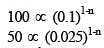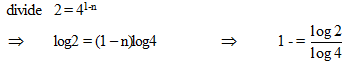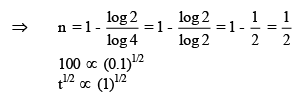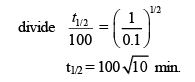QUESTION: 2

### By what factor does the rate of reaction of diacetone alcohol in water solution change if p0H is increased by 2 units other things remaining same ?

Solution:

If [OH-] is decreased by 100 times pOH increases by 2 units. Hence rate decreases by 100 times

QUESTION: 3

### If 60% of a first order reaction was completed in 60 minutes, 50% of the same reaction would be completed in approximately

Solution:
QUESTION: 4

In a reaction, → Product, rate is doubled when the concentration of is doubled, and rate increases by a factor of 8 when the concentrations of both the reactants (Aand B) are doubled, rate law for the reaction can be written as

Solution: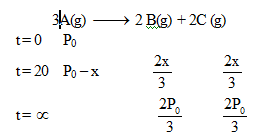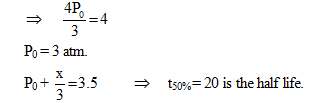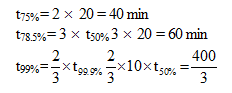*Multiple options can be correct
QUESTION: 5

In the following gaseous phase first order reaction A(g) →2B(g) + C(g) initial pressure was found to be 400 mm of Hg and it changed to 1000 mm of Hg after 20 min. Then :

Solution: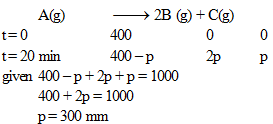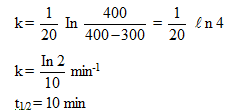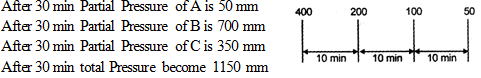*Multiple options can be correct
QUESTION: 6

The polarimeter readings in an experiment to measure the rate of inversion of cane suger (1st order reaction) were as follows time (min) : 0 30 coangle (degree) : 30 20 -15 Identify the true statement (s) log 2 = 0.3, log 3 = 0.48, log 7 = 0.84 , loge 10 = 2.3

Solution: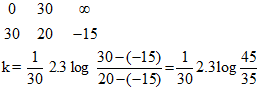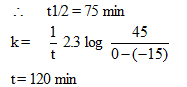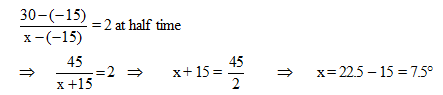*Multiple options can be correct
QUESTION: 7

Identify the true statement(s)

Solution:
*Multiple options can be correct
QUESTION: 8

The half – period T for the decomposition of ammonia on tungsten wire, was measured for different initial pressure P of ammonia at 25°C. Then P (mm Hg) 11 21 48 73 120 T (sec) 48 92 210 320 525

Solution: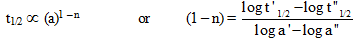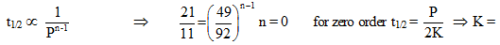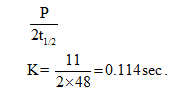QUESTION: 9

The substance undergoes first order decomposition. The decomposition follows two parallel first orde reactions as : If the corresponding activation energies of parallel reaction are 100 and 120 kJ mol-1 then the net activation energy of A is / are :

Solution: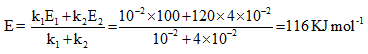*Multiple options can be correct
QUESTION: 10

A certain reaction obeys the rate equation (in the integrated form) [Co -") - Co' - = (n -1) kt where C, is the initial concentration and C is the concentration after time, t.

Solution: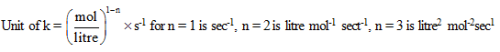*Multiple options can be correct
QUESTION: 11

The ozone layer in the earth's upper atmosphere is important in shielding the earth from very harmful ultraviolet radiation. The ozone, 03, decomposes according to the equation 203(g) -› 302(g). The mechanism of the reaction is thought to proceed through an initial fast, reversible step and then a slow second step.

Step 1: Fast, reversible 03(g)⇔ 02(g) +0(g)

Step 2 : Slow 03(g) + 0(g) → 202(g)

The which of the following is correct ?

Solution:

Slow step is rate determining step, from slow step –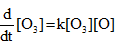Molecularity for step 1 is 1 and for step 2 is 2 as shown in above given reaction.

*Multiple options can be correct
QUESTION: 12

Consider the following case of COMPETING 1ST ORDER REACTIONS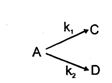After the start of the reaction at t = 0 with only A, the [C] is equal to the [D] at all times. The time in which all three concentrations will be equal is given by

Solution:

K1=K2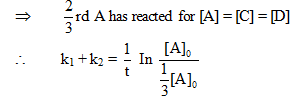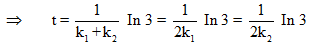*Multiple options can be correct
QUESTION: 13

Rate of a chemical reaction 2A (g) →B (g) is defined as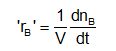Solution: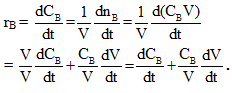QUESTION: 14

Consider the inter conversion of nitrosotnacetoamine into nitrogen phorone and water.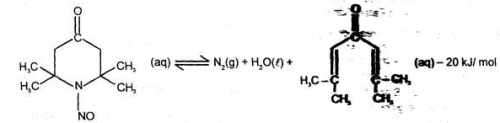The reaction is 1st order in each direction, with an equilibrium constant of 104, the activation energy for the forward reaction is 11usactivation energy for the forward reaction is 57.45 kJ / mol. Assuming arrehemus preexponetial factor of 1012 S–-1

What is the expected forward constant at 300K , it we initiate this reaction starting with only reactant

Solution: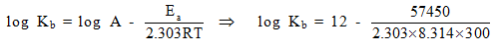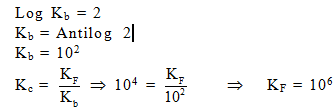QUESTION: 15

Consider the inter conversion of nitrosotnacetoamine into nitrogen phorone and water.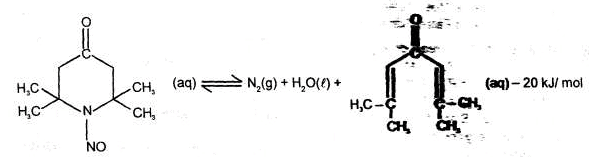The reaction is 1st order in each direction, with an equilibrium constant of 104, the activation energy for the forward reaction is 11usactivation energy for the forward reaction is 57.45 kJ / mol. Assuming arrehemus preexponetial factor of 1012 S–-1

If the change in entropy of the reaction is 0.07 KJ. K-1 mol-1 at 1 atm pressure. Calculate up to which temperature the reaction would not be spontaneous. (For forward reaction)

Solution: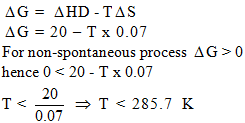QUESTION: 16

Consider the inter conversion of nitrosotnacetoamine into nitrogen phorone and water.The reaction is 1st order in each direction, with an equilibrium constant of 104, the activation energy for the forward reaction is 11usactivation energy for the forward reaction is 57.45 kJ / mol. Assuming arrehemus preexponetial factor of 1012 S–-1

Calculate Kp of the reaction at 300 K

Solution: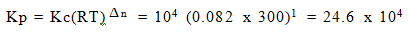QUESTION: 17

Not all chemical reactions proceed to a stage at which the concentrations of the reactants become vanishingly small. Here we consider the kinetics of such reactions.Let a reaction be represented in general terms by the schemewhere k., and k_., represent the rate constants for the forwards and reverse reactions, respectively. The equilibrium constant for this reaction may be written asK = [B]∝ /[A]∝ = K1/K–1                   --(1)where the subscript 00 refers to a time t, sufficiently long to establish equilibrium at the given temperature. The initial concentration of species A is [A]O, and that of B is [B]O . After a time t, let the concentration of species A be [A/t and that of B be [Bat. The total rate of change of [At is given byd[A]t/dt= - K1[A]t + k-1[B]tIf, as is usual, [B]O is initially zero, it follows from a mass balance that at any time t,[Bat = [A]0 - [A]t, whenced[A]t/dt = –K1[A]t + k-1([A]O - [A]t)ord[A]t/dt = – (K1 + k-1) For the reaction  (having both 1ts order reactions), the concentration as a function of time are given for a certain experimental run along with a tangent to the graph at the origin. The ratio of the magnitude of the slopes of the graph of [A/ and [C/ at the origin would be :

Solution: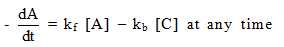And slope between conc and time is known as rate and they always equal at any time.

QUESTION: 18

Not all chemical reactions proceed to a stage at which the concentrations of the reactants become vanishingly small. Here we consider the kinetics of such reactions.
Let a reaction be represented in general terms by the scheme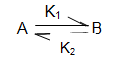where k., and k_., represent the rate constants for the forwards and reverse reactions, respectively. The equilibrium constant for this reaction may be written as

K = [B] /[A] = K1/K–1                   --(1)
where the subscript 00 refers to a time t, sufficiently long to establish equilibrium at the given temperature. The initial concentration of species A is [A]O, and that of B is [B]O . After a time t, let the concentration of species A be [A/t and that of B be [Bat. The total rate of change of [At is given by

d[A]t/dt= - K1[A]t + k-1[B]t
If, as is usual, [B]O is initially zero, it follows from a mass balance that at any time t,

[Bat = [A]0 - [A]t, whence

d[A]t/dt = –K1[A]t + k-1([A]O - [A]t)

or

d[A]t/dt = – (K1 + k-1

At what time is the rate of change of concentration of A equal to rate of change of concentration of C in magnitude ?

Solution: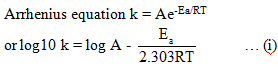Comparing the given equation with equation (i),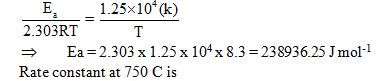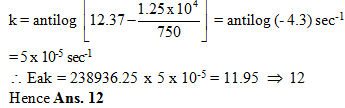QUESTION: 19

There are many reactions which obey a first order rate equation although it reality they are bi- or ter-molecular. As an example of these may be taken the decomposition of Carbonyl sulfide in water, namely, COS + H20 → 002 + H2S
According to the law of mass action this reaction should be second order with the rate dependent on the concentration of both the carbonyl sulfide and the water. Actually however, the rate is found to be first order with respect to the carbonyl sulfide and independent of the water Reactions exhibiting such behaviour are said to be pseudo-molecular.
The pseudo-unimoecuar nature of this reaction is explainable by the fact that water is present in such excess that its concentration remains practically constant during the course of the reaction. Under these condition b x = b, and the rate equation becomes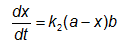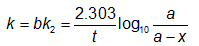which is the equation for a first order reaction. It is evident, however, that the now constant k is not independent of the concentration, as is the case with true first order constants, but may vary with b if the latter is changed appreciably, When such is the case, the true constant k2 can be obtained from k by dividing the latter by b. pseudo-molecular reactions are encountered whenever one or more of the reactants remain constants during the course of an experiment. This is the case with reactions conducted in solvents which are themselves one of the reactants, as in the decomposition of carbonyl sulfide in water, or in the esterification of acetic anhydride in alcohol

(CH3C0)20 + 2C2H5OH → 2CH3C00C2H5 + H20

Again, this is also true of reactions subject to catalysis, in which case the concentration of the catalyst does not change. The decomposition of diacetone alcohol to acetone in aqueous solution is catalysed by  hydroxyl ions, with the rate proportional to the concentration of the alcohol and that of the base. Since the concentration of the base does not change within any one experiment, however, the rate equation reduces to one of first order with respect to the alcohol. But the rate constant k obtained for various concentrations of base are not identical, as may be seen from table. To obtain from these the true second order velocity constant, the k's must be divided by the hydroxyl ion concentration. When this is done excellent k2 values result, as column 3 indicates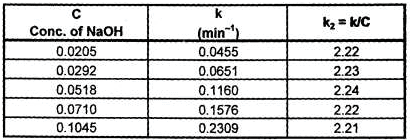Table : Decomposition of diacetone alcohol in water at 25°C (Catalyst : NaOH)

Q.

Which of k or k2 is dependent on concentration ?

Solution:
QUESTION: 20

For the reaction A + B → C ; r = k [A] [B] with [A]0 = 0.001 M and [B]0 = 10 M, the time in which the conc. of A becomes 0.005 M will be nearly

Solution: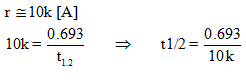QUESTION: 21

Not all chemical reactions proceed to a stage at which the concentrations of the reactants become vanishingly small. Here we consider the kinetics of such reactions.
Let a reaction be represented in general terms by the schemewhere k., and k_., represent the rate constants for the forwards and reverse reactions, respectively. The equilibrium constant for this reaction may be written as

K = [B] /[A] = K1/K–1                   --(1)
where the subscript 00 refers to a time t, sufficiently long to establish equilibrium at the given temperature. The initial concentration of species A is [A]O, and that of B is [B]O . After a time t, let the concentration of species A be [A/t and that of B be [Bat. The total rate of change of [At is given by

d[A]t/dt= - K1[A]t + k-1[B]t
If, as is usual, [B]O is initially zero, it follows from a mass balance that at any time t,

[Bat = [A]0 - [A]t, whence

d[A]t/dt = –K1[A]t + k-1([A]O - [A]t)

or

d[A]t/dt = – (K1 + k-1

What is the Cc of the reaction ​?

Solution: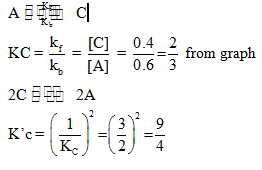QUESTION: 22

There are many reactions which obey a first order rate equation although it reality they are bi- or ter-molecular. As an example of these may be taken the decomposition of Carbonyl sulfide in water, namely, COS + H20 → 002 + H2S
According to the law of mass action this reaction should be second order with the rate dependent on the concentration of both the carbonyl sulfide and the water. Actually however, the rate is found to be first order with respect to the carbonyl sulfide and independent of the water Reactions exhibiting such behaviour are said to be pseudo-molecular.
The pseudo-unimoecuar nature of this reaction is explainable by the fact that water is present in such excess that its concentration remains practically constant during the course of the reaction. Under these condition b x = b, and the rate equation becomeswhich is the equation for a first order reaction. It is evident, however, that the now constant k is not independent of the concentration, as is the case with true first order constants, but may vary with b if the latter is changed appreciably, When such is the case, the true constant k2 can be obtained from k by dividing the latter by b. pseudo-molecular reactions are encountered whenever one or more of the reactants remain constants during the course of an experiment. This is the case with reactions conducted in solvents which are themselves one of the reactants, as in the decomposition of carbonyl sulfide in water, or in the esterification of acetic anhydride in alcohol

(CH3C0)20 + 2C2H5OH → 2CH3C00C2H5 + H20

Again, this is also true of reactions subject to catalysis, in which case the concentration of the catalyst does not change. The decomposition of diacetone alcohol to acetone in aqueous solution is catalysed by  hydroxyl ions, with the rate proportional to the concentration of the alcohol and that of the base. Since the concentration of the base does not change within any one experiment, however, the rate equation reduces to one of first order with respect to the alcohol. But the rate constant k obtained for various concentrations of base are not identical, as may be seen from table. To obtain from these the true second order velocity constant, the k's must be divided by the hydroxyl ion concentration. When this is done excellent k2 values result, as column 3 indicatesTable : Decomposition of diacetone alcohol in water at 25°C (Catalyst : NaOH)

Q.

By what factor does the rate of reaction of diacetone alcohol in water solution change if p0H is increased by 2 units other things remaining same ?

Solution:

If [OH-] is decreased by 100 times pOH increases by 2 units. Hence rate decreases by 100 times.

QUESTION: 23

From the following data answer the questions : Reaction :  A + B → P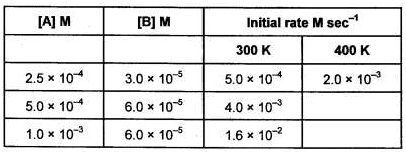The order w. r.t A is

Solution: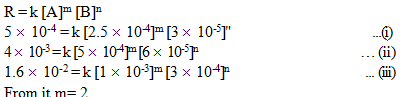QUESTION: 24

From the following data answer the questions : Reaction :  A + B→ P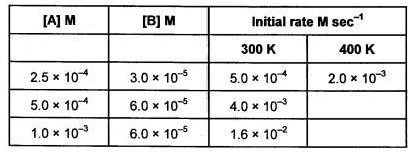The value of rate constant at 300 K is (M-2 sec-1)

Solution: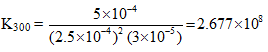QUESTION: 25

From the following data answer the questions : Reaction :  A + B → PThe energy of activation for reaction (KJ/mol) is

Solution: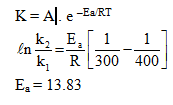QUESTION: 26

The rate law expressed the relationship of the rate of a reaction to the rate constant and the concentration of the reactants raised to some powers for the general reaction aA + bB → cC + dD

Rate law takes the form r = k [A]X [B]y

where x and y are number that must be determined experimentally k is the rate constant and [A] and [B] are concentration of A & B respectively.

Q.

Gaseous reaction A → B + C follows first order kinetics concentration of A changes from 1M to 0.25M in 138.6 min. Find the rate of reaction when conc. of A is 0.1 M.

Solution: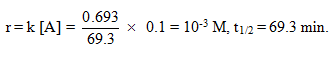QUESTION: 27

The rate law expressed the relationship of the rate of a reaction to the rate constant and the concentration of the reactants raised to some powers for the general reaction aA + bB → cC + dD

Rate law takes the form r = k [A]X [B]y

where x and y are number that must be determined experimentally k is the rate constant and [A] and [B] are concentration of A & B respectively.

Q.

The initial rate of zero order reaction of the gaseous equation A (g) → 2B (g) is 10-2 M min1 if the initial conc. of A is 0.1 M what would be conc. of B after 60 sec.

Solution: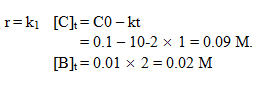QUESTION: 28

The rate law expressed the relationship of the rate of a reaction to the rate constant and the concentration of the reactants raised to some powers for the general reaction aA + bB → cC + dD

Rate law takes the form r = k [A]X [B]y

where x and y are number that must be determined experimentally k is the rate constant and [A] and [B] are concentration of A & B respectively.

Q.

The variation of concentration of 'A' with time in two experiments starting with two different initial concentration of 'A' is given by the following graph.The reaction is represented by A (aq) → B(aq) what is rate of reaction 1.5M (M/min) when conc. of A in aqueous solution was 1.8 M.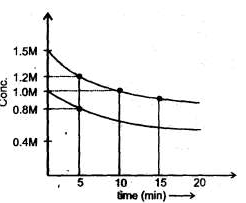Solution: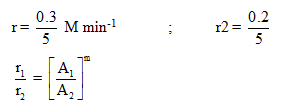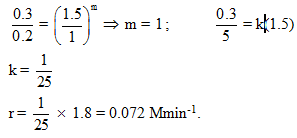QUESTION: 29

For a hypotherical elementary reaction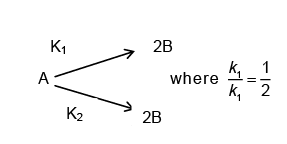.Initially only 2 moles of A are present.

The total number of moles of A, B and C at the end of 50% reaction are:

Solution:

When 1 mole of A is present then 2 mole of B and C will forms after completion of the reaction but when 50% is complete then the total mole = 1 + 2 * 1 = 3.

QUESTION: 30

For a hypotherical elementary reaction.Initially only 2 moles of A are present.

Number of moles of B are :

Solution:

Mole of B = 0.666.

QUESTION: 31

The ionic radii of A+ and B- ions are 0.98 x 10-10 m and 1.81 x10-10 m. The coordination number of each ions in AB is

Solution:

Given, ionic radius of cation (A+) =0.98 x 10-10m
Ionic radius of anion (B-)=1.81 x 10-10m
therefore,
The coordination number of each ion in AB =?
Now we have.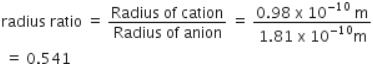If radius ratio range is in between 0.441-0.732 ion would have an octahedral structure with coordination number six.